# Problem: An ideal monatomic gas is contained in a cylinder with a movable piston so that the gas can do work on the outside world, and heat can be added or removed as necessary. The figure shows various paths that the gas might take in expanding from an initial state whose pressure, volume, and temperature are , , and  respectively. The gas expands to a state with final volume . For some answers it will be convenient to generalize your results by using the variable , which is the ratio of final to initial volumes (equal to 4 for the expansions shown in the figure.)The figure shows several possible paths of the system in the pV plane. Although there are an infinite number of paths possible, several of those shown are special because one of their state variables remains constant during the expansion. These have the following names:Adiabiatic: No heat is added or removed during the expansion.Isobaric: The pressure remains constant during the expansion.Isothermal: The temperature remains constant during the expansion.Calculate the work WC done by the gas during the isothermal expansion.Express WC in terms of p0, V0, and RV

###### FREE Expert Solution

During an isothermal expansion, the work done by the gas is expressed as:

$\overline{){\mathbf{W}}{\mathbf{=}}{{\mathbf{\int }}}_{{\mathbf{v}}_{\mathbf{0}}}^{{\mathbf{v}}_{\mathbf{f}}}{\mathbf{P}}{\mathbf{d}}{\mathbf{V}}}$

Also,

$\overline{){\mathbf{P}}{\mathbf{V}}{\mathbf{=}}{\mathbf{n}}{\mathbf{R}}{\mathbf{T}}}$

84% (99 ratings)###### Problem Details

An ideal monatomic gas is contained in a cylinder with a movable piston so that the gas can do work on the outside world, and heat can be added or removed as necessary. The figure shows various paths that the gas might take in expanding from an initial state whose pressure, volume, and temperature are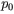,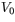, and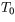respectively. The gas expands to a state with final volume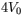. For some answers it will be convenient to generalize your results by using the variable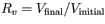, which is the ratio of final to initial volumes (equal to 4 for the expansions shown in the figure.)

The figure shows several possible paths of the system in the pV plane. Although there are an infinite number of paths possible, several of those shown are special because one of their state variables remains constant during the expansion. These have the following names:

• Adiabiatic: No heat is added or removed during the expansion.
• Isobaric: The pressure remains constant during the expansion.
• Isothermal: The temperature remains constant during the expansion.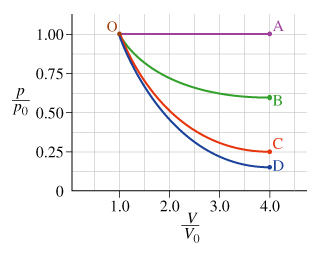Calculate the work WC done by the gas during the isothermal expansion.
Express WC in terms of p0, V0, and RV

Frequently Asked Questions

What scientific concept do you need to know in order to solve this problem?

Our tutors have indicated that to solve this problem you will need to apply the Work & PV Diagrams concept. You can view video lessons to learn Work & PV Diagrams. Or if you need more Work & PV Diagrams practice, you can also practice Work & PV Diagrams practice problems.